### Straight Channel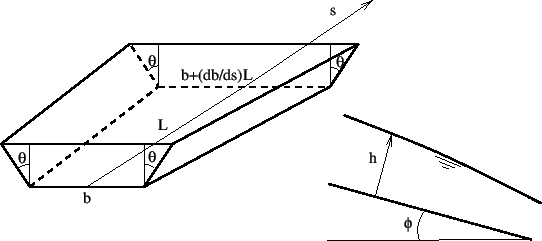The straight channel is characterized by a trapezoid cross section, the bottom width of which can be defined to vary linearly. This is illustrated in Figure 119. The following constants have to be specified on the line beneath the *FLUID SECTION,TYPE=CHANNEL STRAIGHT card:

• the width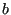• the slope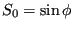(if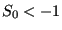the slope is calculated from the coordinates of the end nodes belonging to the element)
• the length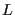• the trapezoid angle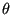• the grain diameter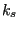for the White-Colebrook law or the Manning constant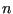for the Manning law (in the latter case the user has to specify the parameter MANNING on the *FLUID SECTION card)
• the number of the upstream reference element

Example files: channel1, chanson1.